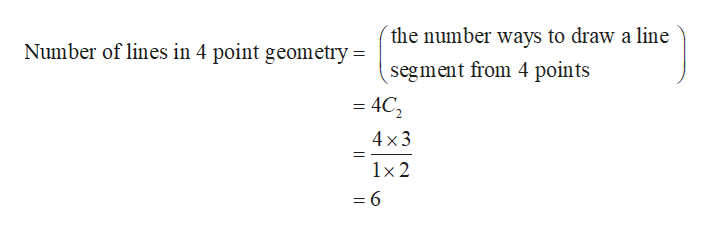# prove Theorem 4P.1Theorem 4P.1There are exactly six lines in the four-point geometry.

Question
2 views

prove Theorem 4P.1

Theorem 4P.1

There are exactly six lines in the four-point geometry.

check_circle

Step 1

The known fact is that in four point geometry, any two distinct points have exactly one line on both of them and each line is o...help_outlineImage Transcriptionclosethe number ways to draw a line Number of lines in 4 point geometry = segment from 4 points = 4C2 4 x3 1x 2 =6 fullscreen

### Want to see the full answer?

See Solution

#### Want to see this answer and more?

Solutions are written by subject experts who are available 24/7. Questions are typically answered within 1 hour.*

See Solution
*Response times may vary by subject and question.
Tagged in

### Coordinate Geometry